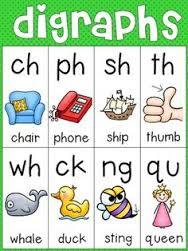Digraphs
3 years ago
jhami
Save
Edit
Host a game
Live GameLive
Homework
Solo Practice
Practice15 QuestionsShow answers
• Question 1
30 secondsQ. What is the chunk?
ch
sh
bl
wh
• Question 2
30 secondsQ. What is the chunk?
br
fl
pl
st
• Question 3
30 secondsQ. What is the chunk?
ch
sh
pl
th
• Question 4
30 secondsQ. What is the chunk?
th
st
wh
ch
• Question 5
30 secondsQ. What is the chunk?
fl
ch
sh
th
• Question 6
30 secondsQ. What is the chunk?
sh
ch
wh
th
• Question 7
30 secondsQ. What is the chunk?
ch
th
wh
sh
• Question 8
30 secondsQ. What is the chunk?
ch
sh
wh
th
• Question 9
30 secondsQ. What is the chunk?
ch
sh
wh
th
• Question 10
30 secondsQ. What is the chunk?
fl
bl
pl
st
• Question 11
30 secondsQ. What is the chunk?
pl
bl
cl
sl
• Question 12
30 secondsQ. What is the chunk?
bl
cl
pl
sl
• Question 13
30 secondsQ. What is the chunk?
pl
bl
sl
cl
• Question 14
30 secondsQ. What is the chunk?
cl
cr
bl
th
• Question 15
30 secondsQ. What is the chunk?